# Google Sheets percentage formulas - usage and examples

You may think percentage calculations are useful only if you use them for work. But in reality, they help you in everyday life. Do you know how to tip properly? Is this discount a real deal? How much will you pay with this interest rate? Come find the answers to these and other similar questions in this article.

## What is percentage

As you most likely already know, percent (or per cent) means one-hundredth part. It is marked by a special sign: %, and represents a part of the whole.

For example, your and your 4 friends are getting a birthday gift for another friend. It costs \$250 and you're chipping in together. What percent of the total you're investing in the present?

This is how you usually calculate percents:

(Part/Total)*100 = Percentage

Let's see: you're giving away \$50. 50/250*100 – and you get 20% of the gift cost.

However, Google Sheets makes the task simpler by calculating some parts for you. Below I'll show you those basic formulas that will help you get different results depending on your task, whether calculating percentage change, percentage of the total, etc.

## How to calculate percentage in Google Sheets

Part/Total = Percentage

Unlike the previous formula, this one doesn't multiply anything by 100. And there's a good reason for that. Simply set the format of cells to percent and Google Sheets will do the rest.

So how will this work on your data? Imagine you keep track of ordered and delivered fruits (columns B and C respectively). To calculate the percentage of what's been received, do the following:

• Enter the below formula to D2:

`=C2/B2`

• Copy it down your table.
• Go to Format > Number > Percent in Google Sheets menu to apply the percentage view.

Note. You'll need to go over these steps to create any percentage formula in Google Sheets.

Tip. You can decrease and increase decimal places if necessary using the options from the Google toolbar: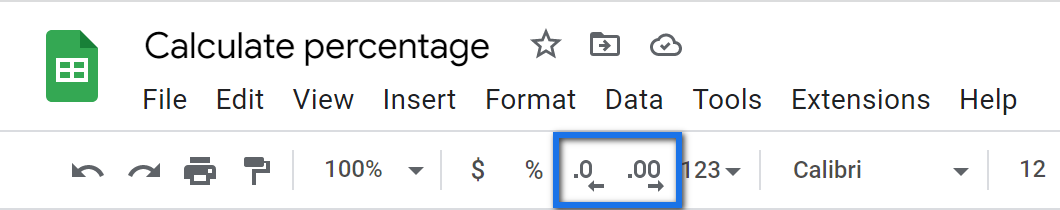Here's how the result looks on real data: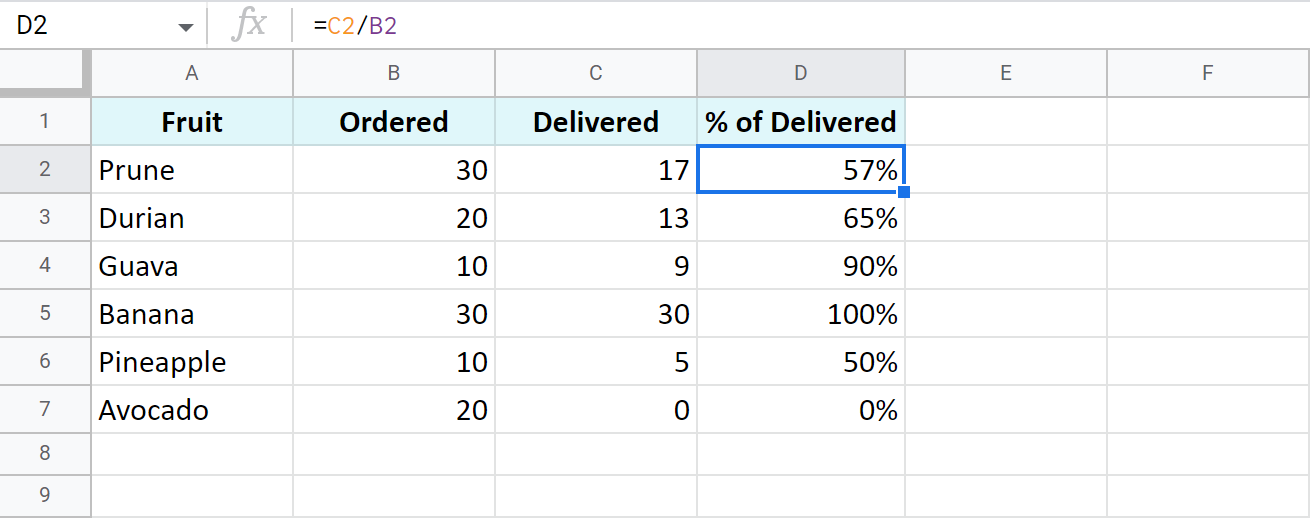I removed all decimal places making the formula show the result as a rounded percent.

Here are a few more examples of calculating percentage of a total. Though the previous shows the same, it works great for that example but may not be enough for other data set. Let's see what else Google Sheets offers.

### A common table with a total at its end

I believe this is the most common case: you have a table with values in column B. Their total resides at the very end of the data: B8. To find the percentage of the total for each fruit, use the same basic formula as before but with a slight difference – an absolute reference to the cell with the total sum.

This type of reference (absolute, with a dollar sign) doesn't change when you copy the formula to other cells. Thus, each new record will be calculated based on the sum in \$B\$8:

`=B2/\$B\$8`

I also formatted the results as percent and left 2 decimals to display: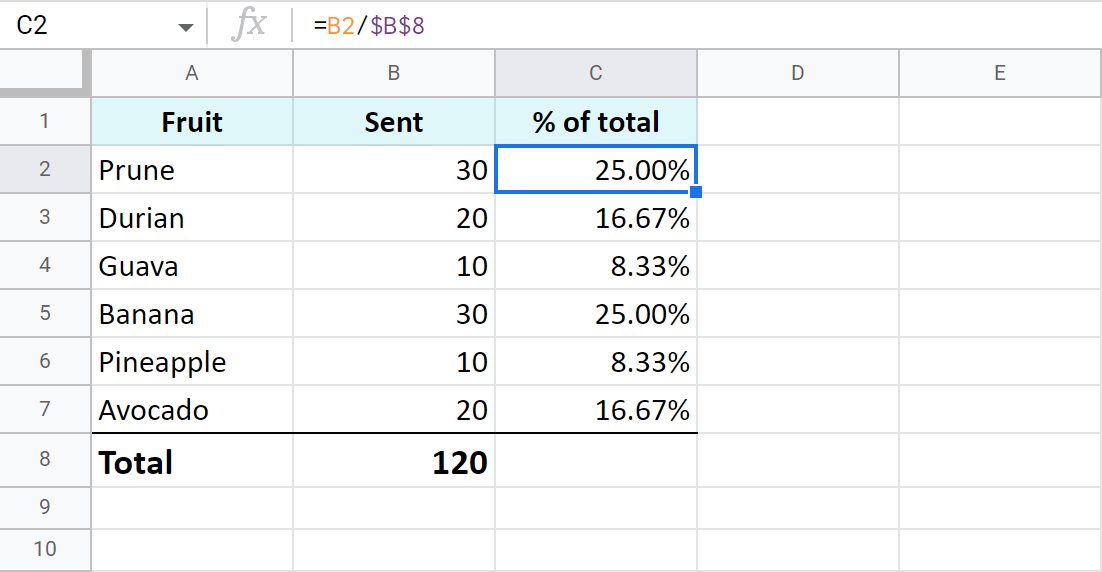### One item takes a few rows – all rows are part of the total

Now, suppose a fruit appears more than once in your table. What part of the total is composed of all deliveries of that fruit? The SUMIF function will help answer that:

=SUMIF(range, criteria, sum_range) / Total

It will sum only numbers belonging to the fruit of interest and divide the result by the total.

See for yourself: column A contains fruits, column B – orders for each fruit, B8 – the total of all orders. E1 has a drop-down list with all possible fruits where I chose to check the total for Prune. Here's the formula for this case:

`=SUMIF(A2:A7,E1,B2:B7)/\$B\$8`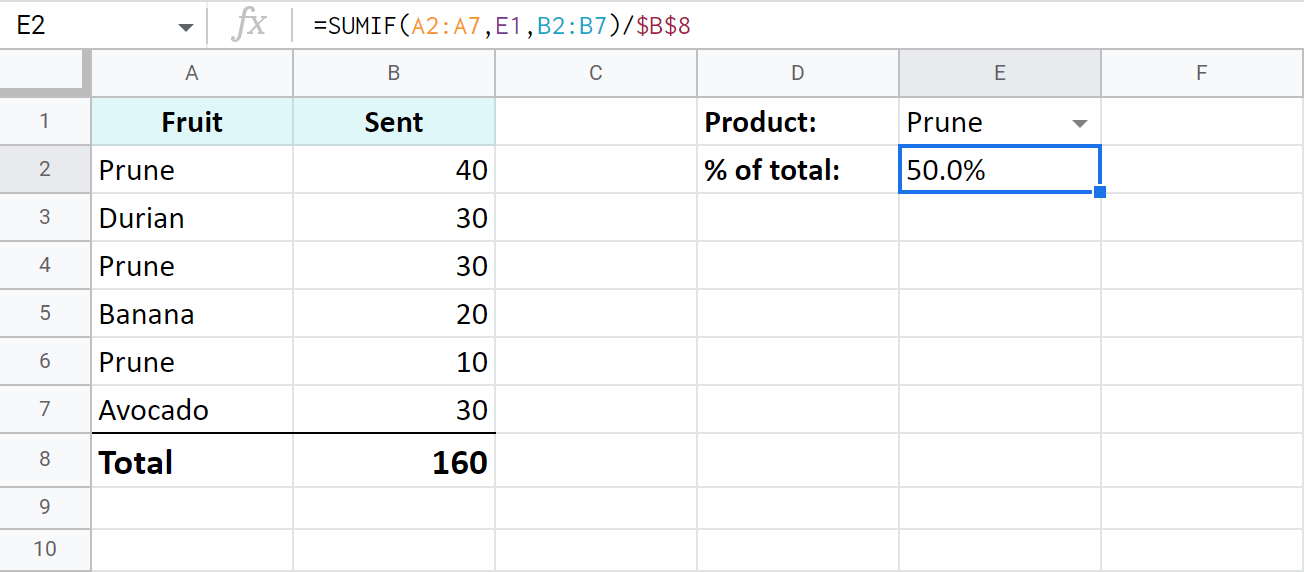Tip. Having a drop-down with fruits is completely up to you. Instead, you can put the necessary name right to the formula:

`=SUMIF(A2:A7,"Prune",B2:B7)/\$B\$8`

Tip. You can also check a part of the total made by different fruits. Just add up a few SUMIF functions and divide their result by the total:

`=(SUMIF(A2:A7,"prune",B2:B7)+SUMIF(A2:A7,"durian",B2:B7))/\$B\$8`

## Percentage increase and decrease formulas

There's a standard formula you can use to calculate percent change in Google Sheets:

`=(B-A)/A`

The trick is to figure out which of your values belong to A and to B.

Let's assume you had \$50 yesterday. You have saved \$20 more and today you have \$70. This is 40% more (increase). If, on the contrary, you've spent \$20 and have only \$30 left, this is 40% less (decrease). This deciphers the formula above and makes it clear which values should be used as A or B:

=(New value – Old value) / Old value

Let's see how this works in Google Sheets now, shall we?

### Work out percent change from column to column

I have a list of fruits (column A) and I want to check how prices have shifted in this month (column C) compared to the previous one (columns B). Here's the percent change formula I use in Google Sheets:

`=(C2-B2)/B2`

I also used conditional formatting to highlight cells with percent increase with red and percent decrease with green: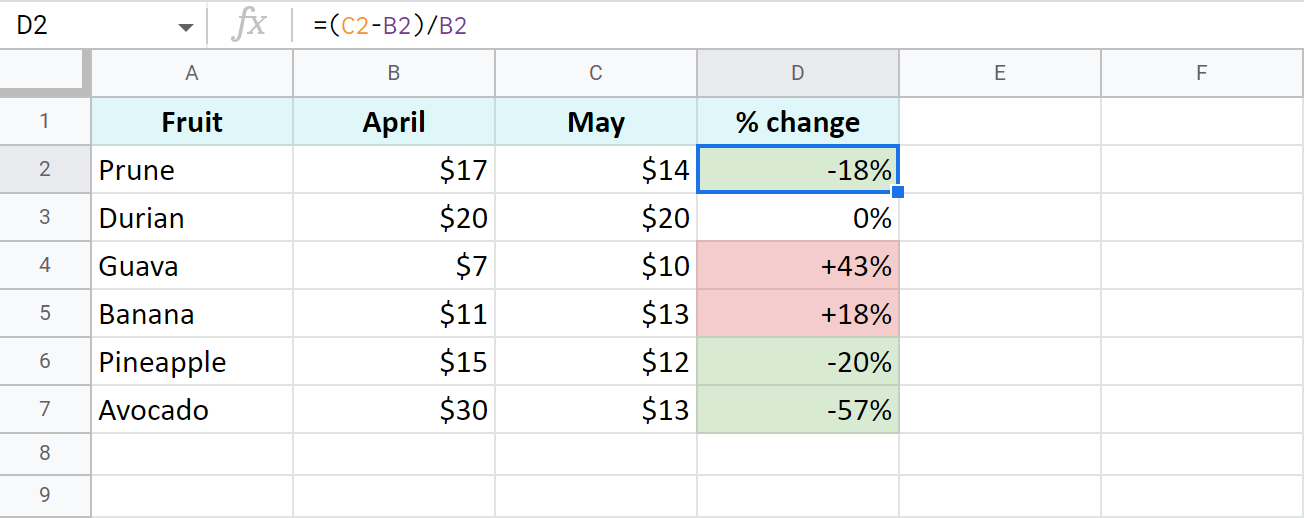### Percent change from row to row

This time, I'm tracking total sales (column B) over each month (column A). To make sure my formula works correctly, I should start entering it from the second row of my table – C3:

`=(B3-B2)/B2`

Copy the formula over all rows with data, apply the percent format, decide on the number of decimals, and voila: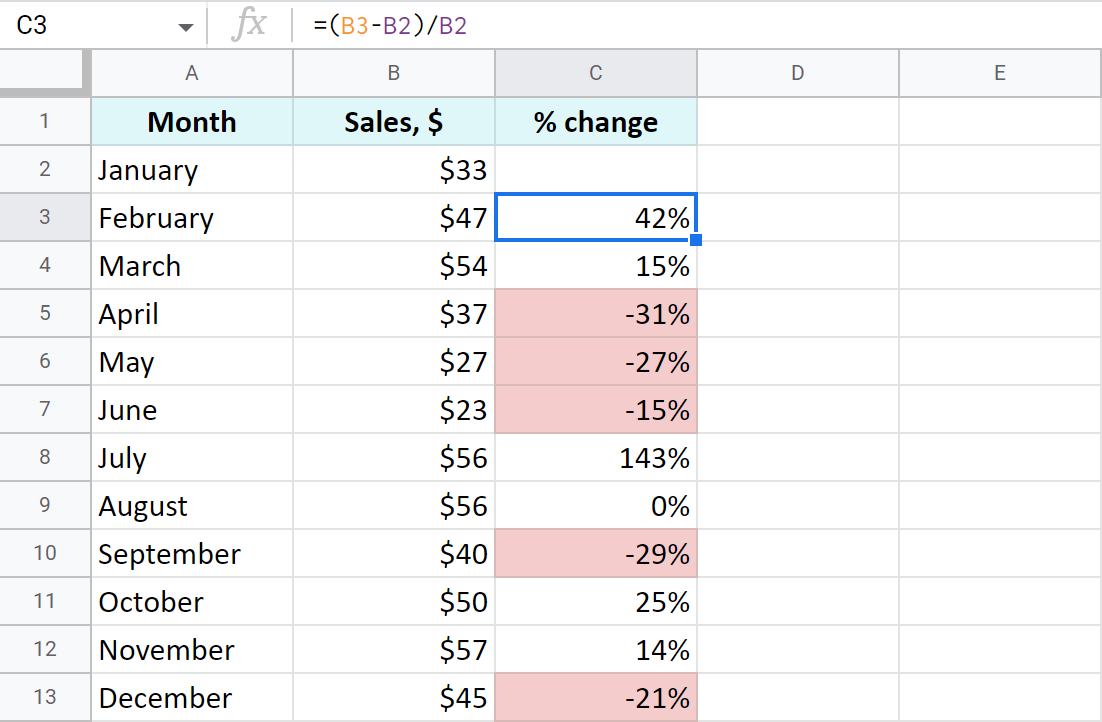Here I also coloured percentage decrease with red.

### Percent change compared to one cell

If you take the same sales list and decide to calculate percentage change based on January only, you will have to always refer to the same cell – B2. For that, make the reference to this cell absolute instead of relative so it doesn't change after copying the formula to other cells:

`=(B3-\$B\$2)/\$B\$2`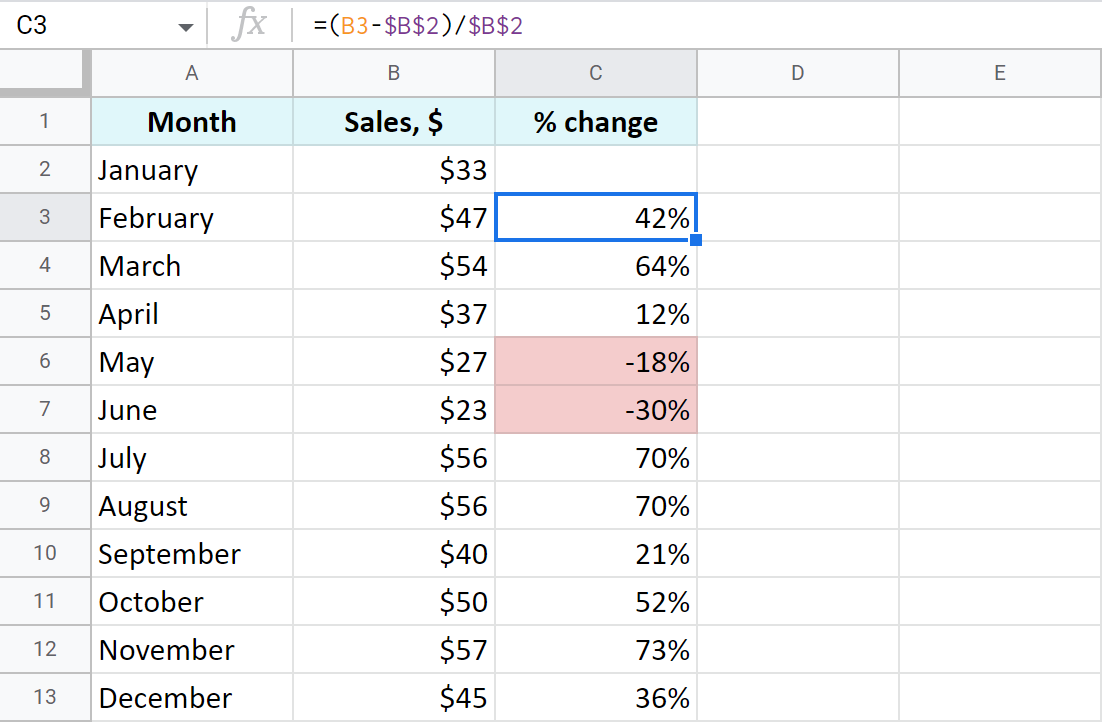Now that you've learned how to operate percentages, I hope getting total and the amount will be a child's play.

### Find amount when having total and percentage

Let's imagine you've spent \$450 shopping abroad and you'd like to have the taxes returned – 20%. So how much exactly should you expect to receive back? How much is 20% of \$450? Here's how you should count:

Amount = Total*Percentage

If you put the total to A2 and the percent to B2, the formula for you is:

`=A2*B2`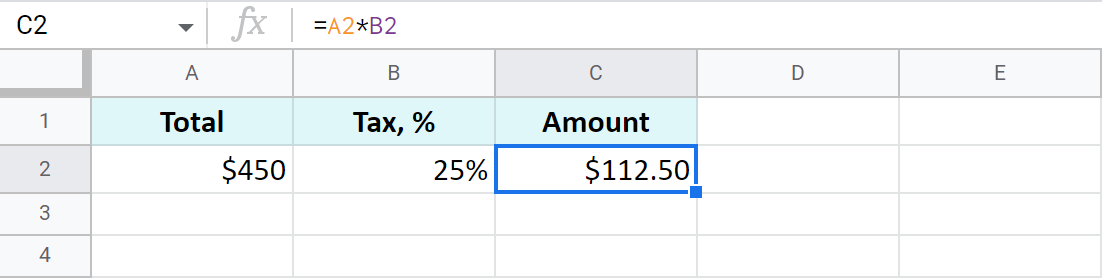### Find total if you know amount and percentage

Another example: you've found an ad where a used scooter is being sold for \$1,500. The price already includes a pleasant 40% discount. But how much would you need to pay for a new scooter like that? The below formula will do the trick:

Total=Amount/Percentage

As the discount is 40%, it means you are to pay 60% (100% – 40%). With these numbers at hand, you can work out the original price (total):

`=A2/C2`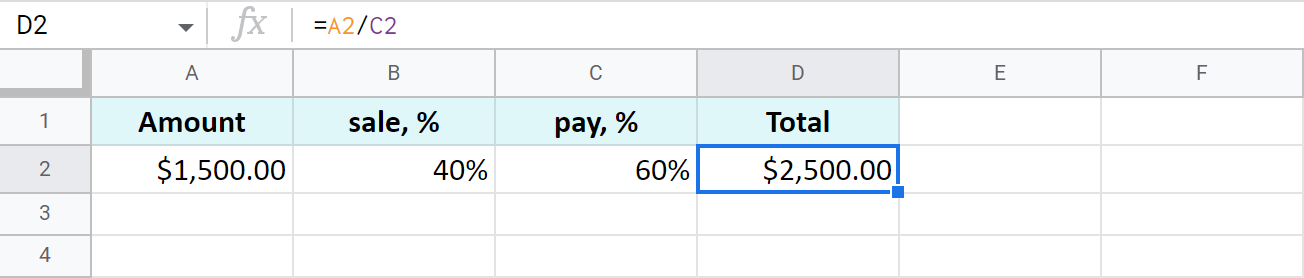Tip. As Google Sheets stores 60% as one hundredth – 0.6, you can get the same result with these two formulas as well:

`=A2/0.6`
`=A2/60%`

## Increase and decrease numbers by percentage

The following examples represent the formulas you may need a bit more often than other ones.

### Increase a number in a cell by percent

A general formula to calculate the raise by some percent is as follows:

=Amount*(1+%)

If you have some amount in A2 and you need to increase it by 10% in B2, here's your formula:

`=A2*(1+B2)`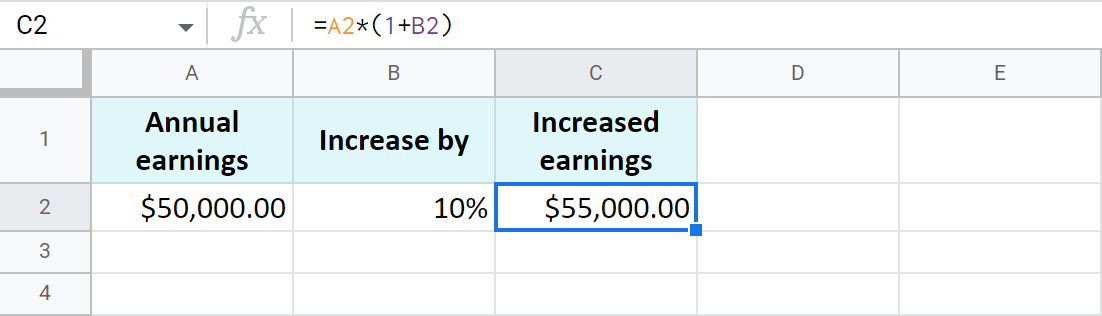### Decrease a number in a cell by percent

To make the opposite and decrease the number by a percent, use the same formula as above but replace the plus sign with a minus:

`=A2*(1-B2)`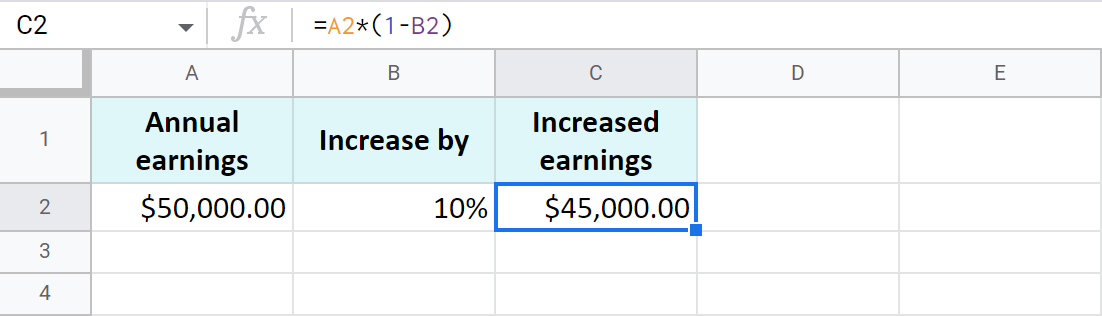### Increase and decrease a whole column by percent

Now assume you have lots of records written in a column. You need to raise each of them by a percentage in that same column. There's a quick way (6 steps to be exact) to do that with our Power Tools add-on:

1. Select all values you'd like to raise first and apply the automatic format: Format > Number > Automatic: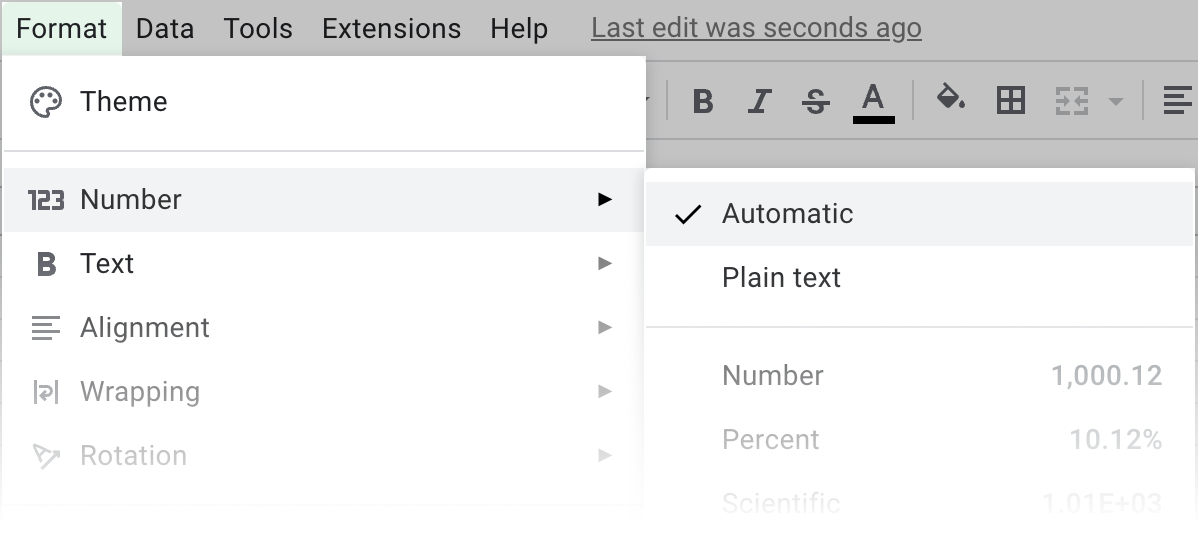Note. This is required to avoid problems with formats on the next steps.

2. Keep the values selected and run the Text tool from Extensions > Power Tools > Tools > Text: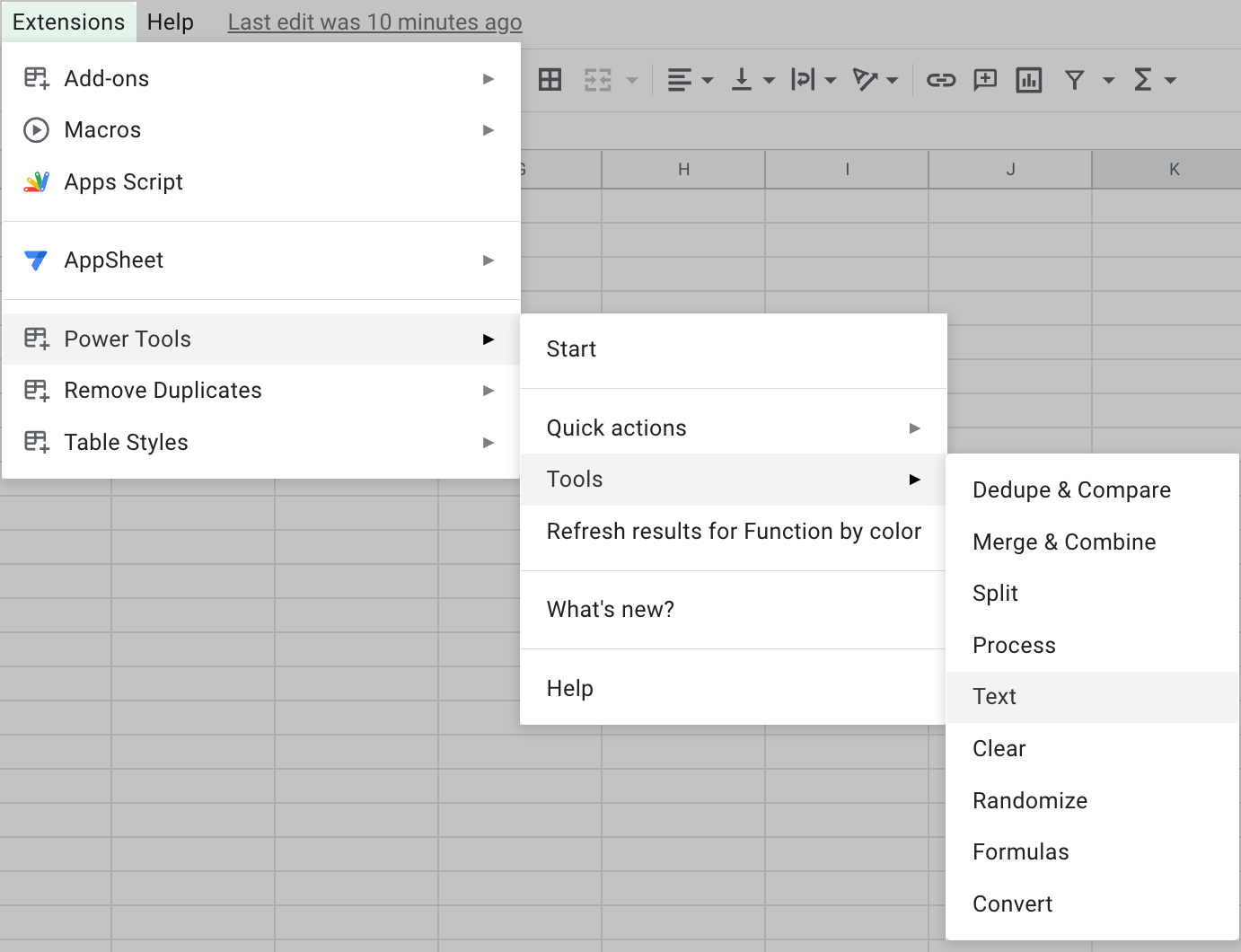3. Open Add text by position and add an equal sign (=) to add it at the beginning of each cell: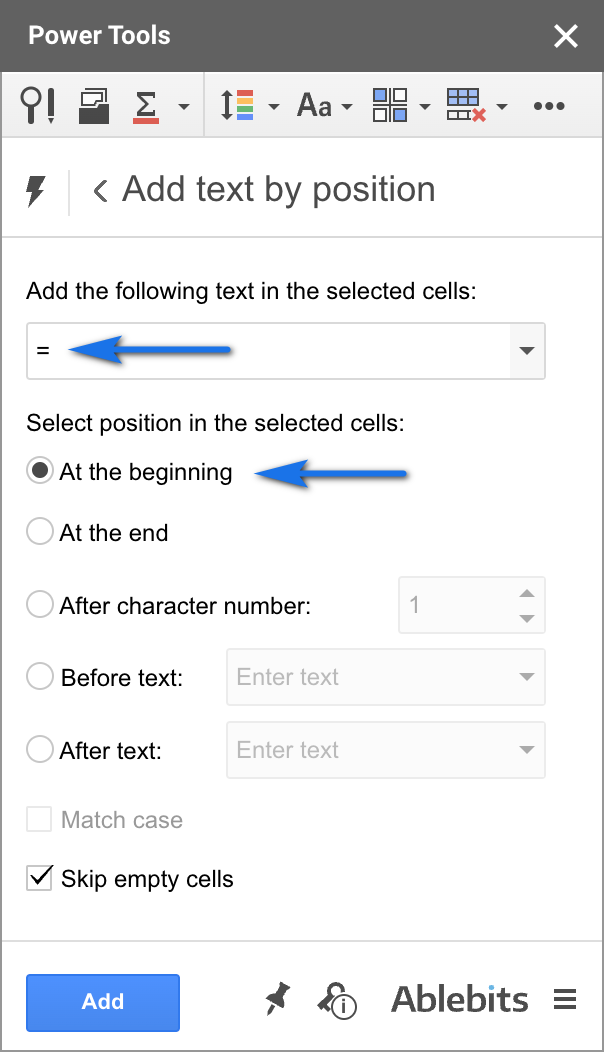4. Click Run to turn all your numbers to formulas: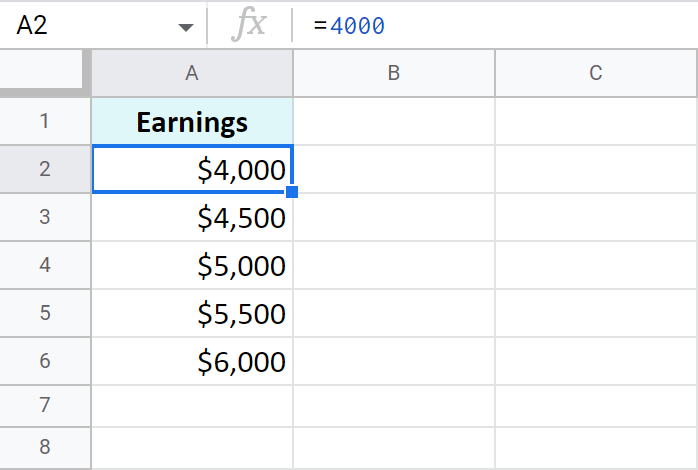5. Proceed to the Formulas tool in Power Tools and select the option to modify all selected formulas.

You will see %formula% is already written there. You are to add those calculations you want to apply to all formulas at once.

Remember the formula to increase a number by percent?

=Amount*(1+%)

Well, you already have those amounts in column A – this is your %formula% for the tool. Now you should only add the missing part to calculate the increase: *(1+10%). The whole entry looks like this:

`%formula%*(1+10%)`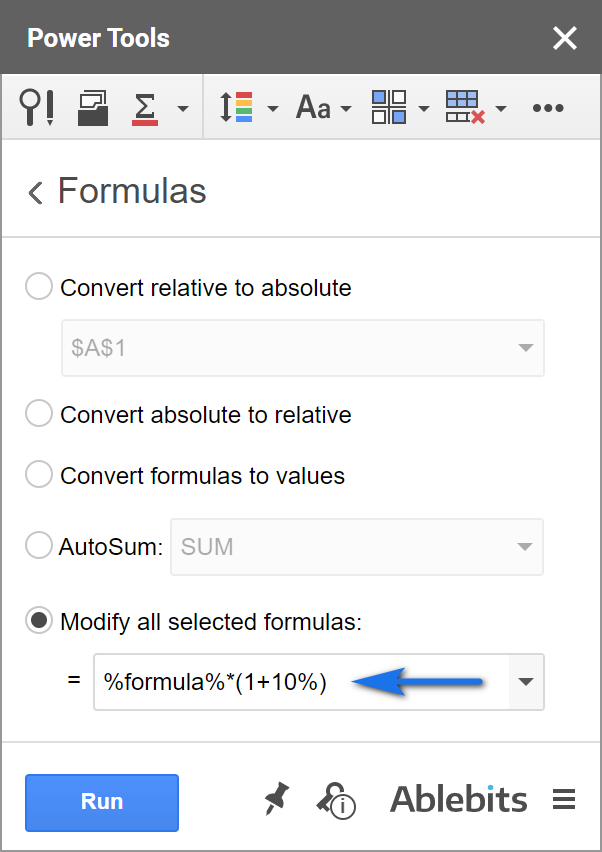6. Hit Run and all records will be raised by 10% at once: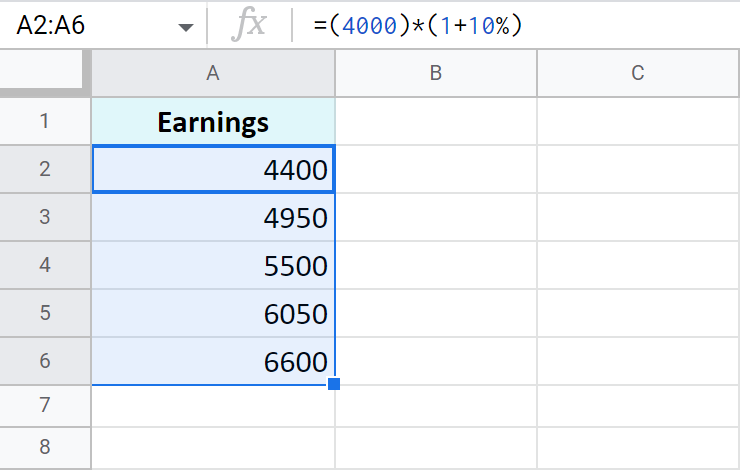Tip. Feel free to apply any number format back to these formulas.

That's it! All these examples are easy-to-follow and are intended to remind those of you who've forgotten or show those who don't know the basic rules of calculating percentage in Google Sheets.

## You may also be interested in

1. I am trying to figure out and "if equal to or less than "amount" multiply by 6.5% of cell value and if greater than "amount" multiply by 8.5% of cell value. example:

If cell F1 is equal to or less than \$40,000, multiply the amount input into cell F1 by 6.5%, but if cell F1 is greater than \$40000, multiply the amount input in cell F1 by 8.5%

• Hello Daniel,

What you describe is what the IF function does. Check out this tutorial to learn how to build its formulas.

2. I'm trying to figure out how to set each cell in column B to calculate percentage from each adjoining cell in column A, using 1's for correct answer and 0's for incorrect answers, so looking for percentage of correct answers. Can you help please? Example below:
Column A Column B
10110001000 Need % from adjoining cell in column A
0011111001110 Need % from adjoining cell in column A

• Hello Michael,

I'm sorry but your task is not clear. Please consider sharing an editable copy of your sample spreadsheet with us (support@apps4gs.com) and include the result you'd like to get. I kindly ask you to shorten the tables to 10-20 rows.
Note. We keep that Google account for file sharing only and don't monitor its Inbox. Please do not email there. Once you share the file, just confirm by replying to this comment.

3. I am looking to get percentages for individuals, the number of jobs they’ve done and the number of incidents.

Joe measured 10 jobs (B1 name)
Out of 10 jobs (using check box) 5 had incidents (C1 check box)
Joe has a 50% incident rate (D1 percentage)

I hope I am asking this question accurately

• Hello Zac,

Have you tried this formula? If it doesn't work as you need, please consider sharing an editable copy of your sample spreadsheet with us (support@apps4gs.com) and include the result you'd like to get & your formula.
Note. We keep that Google account for file sharing only and don't monitor its Inbox. Please do not email there. Once you share the file, just confirm by replying to this comment.

4. Hello! I have a bit of a complicated one. Is there a way to have a formula that can calculate a score based on certain criteria being checked off?

A3: Drop Down Menu (option A - 0%, option B - 10%, option C - 20%, option D - 30%)
B3: Check Box - 20% if checked
C3: Check Box - 20% if checked
D3: Check Box - 5% if checked
E3: Check Box - 25% if checked
F3: total score

the criteria being if the drop down menu has option D selected, and all boxes checked, that would be a score of 100%. Then of course, the score would change depending on what is checked or which drop down option applies.

So is there a formula that can do this? I know it's a little complicated!!

5. How would I calculate this--

What percentage of the values in this column are less than ________?

6. Hi, I'm a little stuck and hoping you can help. Lets say in column A I have a list of names (person 1, person 2, person 3, person 1, person 2, person 3) which appear multiple times and not in the same order, and Column B states YES or NO next to each name. How can I calculate the percentage of YES or NO for each person if the names appear multiple times in Column A? Thanks!

• Hi Rebecca,

To find percentage of a total, you need to divide the number by total.

The total here is all occurrences of each name: =COUNTIF(A2:A10,A2)
Where A2 is person 1.

The amount of YES next to person 1:
=COUNTIFS(A2:A10,A2,B2:B10,"yes")

The percentage of YES:
=COUNTIFS(A2:A10,A2,B2:B10,"yes")/COUNTIF(A2:A10,A2)

You count all NO for person 1 in a similar manner.
To count this for other people, change A2 accordingly.

Functions used in the formula:
COUNTIF
COUNTIFS

• Thanks SO much for the fast response. This is exactly what I needed!

• You're most welcome, Rebecca, glad I could help! :)

7. I have a column of numbers. I'd like to be able to find out the percentage of how many times the numbers in this list are greater than 0.

Example:

0
0
4
-1

This would be 25%.

Is there a formula for this?

• Hello Jeanne,

Yes, here you go (assuming these values are in B2:B5):
=COUNTIF(B2:B5,">0")/COUNT(B2:B5)

Apply the Percent format to the resulting cell as described in this part of the article.

Functions in use:
COUNTIF
COUNT

8. I need to make a column of percentages based on a column of fractions. So my column C has fractions (example: 20/43) and I want column D to show thoses in percentages.

• Hello Elizabeth,

If I understand your task correctly, you should be able to calculate that by following the steps in this part of the article.

9. Hello, I would like to capture the percentage of three numbers (represents three year totals) to see a trend either negative or positive.

Thank you,

Brian

• Hello Brian,

For me to be able to help you, please describe the task in detail. Provide examples of the data you have and specify how you want it to look as a result. Thank you.

• Hello,
I am working with year-to-date statistics over a three-year period covering 2021, 2022, 2023. Example: 2021= 40 2022= 45 and 2023= 35. I would like to reflect a positive or negative % over the three year period. I used this function =TO_PERCENT((Q29-Z27)/Z27) to calculate 2022 and 2023 and it reports -22% which is perfect. Except I need it for three years, I’m not sure how to include 40 from 2021 into the equation. The total for 2021 is displayed in H29 with the percentage result being displayed on the same sheet in Z29

Your expertise is certainly appreciated by many.

• Hello Brian,

Thank you for the details. So you need to calculate the percent change between 2023 and 2021, correct?

• Hello,

Correct, showing a % trend. Is 2023 higher or lower than 2021 & 2022

Thank you again.

• If you're trying to compare 2023 with 2021 & 2022, you will need 2 formulas:

1. one for 2022 vs 2023: =TO_PERCENT((B4-B3)/B3)
2. and another for 2021 vs 2023: =TO_PERCENT((B4-B2)/B2)

If you need both results in the same cell, you will have to concatenate the calculations:
=ROUND((B4-B3)/B3*100)&" & "&ROUND(((B4-B2)/B2)*100)

If I'm missing something and this is not what you want, please specify the result you expect to get. Thanks!

10. Hi,
I was wondering if you can make a progress bar, once a certain numbers of cells are checked pr have a certain color.
Thank you

11. Hi,

I need to figure out the following.

Example:
I need the total from row 1 plus the total from row 2 plus just 30% of the total from row 3, how would this be done?

Thanks

12. Hi Natalia,

I do have a question i can't seem to find the answer to though, if you could point me in the right direction i'd be forever grateful to you!

I'm building out a material spreadsheet for my plumbing company, I have a cell that is designated for my cost from supplier. My material markup is based on a sliding range meaning the higher the cost, the less my markup percentage will be.

For example,
from \$.01-\$5 the markup will be 4x
from \$5.01-\$10 the markup will be 3.25x
and so on...

Is there a way to set up a few different formulas based on different cost ranges and different percentages to calculate my selling price? This spread sheet is roughly 1400 items and my cost gets updated once every two months so going through each line item would take forever.

If you have any insight on how to do this or what you feel would be the best way to go about it i'd greatly appreciate it.
Thank you!

• Hi Devin,

If I understand your task correctly, you need to use the IF function. It will check the cost and calculate the percentage accordingly.

13. Whats the formula of

plus 1% per 14

• Hello,

• If I were to make a formula in excel how can I come up with it?

If I put X I would like to know how many percent it is in total. If 14 is equal to 1%

• If I understand you correctly, this formula will help:
=(B3*C2)/B2

Where B3 is your X, C2 is 1%, B2 is 14.

• It works perfectly! You're good at this and thank you for your help Natalia! More power to your ablebits team

• My pleasure! Appreciate your lovely words :)

14. I am using a formula to add 2 percentages =1.45+2.41. However, when it completes the formula it gives me 386.00% instead of 3.86%. Right now I'm dividing by 100 to get the correct number, but I've never had this happen before. What could cause this?

• Hello Sarah,

This happens because 1.45 and 2.41 are numbers. They total in 3.86 that you format as percent. This turns them into 386.00%

I'd advise you to enter 1.45 and 2.41 to separate cells and format those cells as percent first. Then sum them up. Otherwise, dividing the total by 100 is a correct solution.

15. Hello,

I have averages from scores that I need to combine into a range and then create a chart with three different x-axis data points for numbers in the defined ranges. On the y-axis, I need the percentage out of 100%. Example (I have 100 scores with averages from 0-5. Scores that fall between 0-3 = never, 3-3.49 = sometimes, 3.5-5 = often). I need the chart to group all of the scores for those three ranges (x-axis), and I need the percentages on the y-axis to be out of all 100 scores

• Hello Green,

Alternatively, please consider sharing a small sample spreadsheet with us (support@apps4gs.com) with 2 sheets: (1) a copy of your source data (2) the result you expect to get. The result sheet is of great importance as it gives us a better understanding than any text description. I kindly ask you to shorten the tables to 10-20 rows. I'll look into it.

Note. We keep that Google account for file sharing only and don't monitor its Inbox. Please do not email there. Once you share the file, just confirm by replying to this comment.

16. Hello, this is a helpful tutorial, but I'm not finding what I need.
Is there a way to turn a decimal into a payment factor?

Example:
6.5 into 0.065
10 into 0.10
9.75 into 0.0975

Any insight would be appreciated

• Hello Erik,

I'm not sure about your whole task but from what I can see, I can suggest dividing by 100.

17. Hi - the formula for percentage increase/decrease is working perfectly for the majority of my data, but some of the numbers involved are zeros, which brings up an error as you can't divide by zero. Is there any way to fix this?
e.g.
2021 = 0
2022 = 13
How can I calculate the percentage increase from 2021 o 2022 when the =(2022 value - 2021 value) / 2021 value brings up a #DIV/0 error?

• Hi Emily,

This depends on what you want to see as a result. In both cases it's the IFERROR function that will help you. Please see the formula examples here (the article is for Excel but it works the same in Google Sheets).

18. hello ma’am. if i have over 10 columns with checkboxes, how do i make the total of these columns equals to 100%? in my example, i have 13 columns and the total is 130% when i check all the boxes.

• Hello ariana,

Use a formula like this:
=COUNTIF(A1:M10,TRUE)/COUNTA(A1:M10)

Make sure to format a cell with the formula as Percent.

19. Hey,
I was just wondering how you would get google sheets to show something out of a total. for example, I have checkboxes in separate cells and if I check say 3 out of 10 of the boxes, is there a formula to show that in a separate cell? (eg. 3/10) So if I checked another box, it would automatically change instead of having to change it manually.
Thanks

• Hey Darsha,

Assuming your checkboxes are in column A, use this formula:
=COUNTIF(A2:A11,TRUE)&"/10"

20. Dear Sir,
I got a formula from here, I really appreciate you for these formulas.

Thank you very much.

• You're most welcome, Akbar!

21. Hi;

Thanks for this post. I am trying to implement this tax bracket as a formula for my family Budget Spreadsheet- I need this >>>( \$9,615 plus 22% of the amount over \$83,550) .. can someone help me write this formula to add to my yearly income.

• Hi Emanuel,

Assuming \$9,615 is in A2 and \$83,550 is in B2, here's a formula:
=A2+IF(B2>83550,B2*0.22,"")

22. Hello I'm wondering if anyone knows the formula

I want to be able to type a monetary amount into a single cell and have it decreased by 20% In that cell
For example
I want to type \$100 but it automatically deduct 20% of that and shows the deduction in the same cell not the \$100
Is that possible?

• Hello Lori,

Due to the nature of Google Sheets, you can't enter a value into a cell and have a working formula in that cell at the same time. It's either one or another I'm afraid. Hence, for your task, you need to have two columns (they can be on different sheets): one with the value to enter, another with a formula to decrease that value by percent.

23. I am new to SHEETS and need help with the following. I have searched for hours and can only figure out half of what I need.

If the Value of C13 is 20% OR HIGHER than the value of C20 = Red (I have this part figured out with =C13>=.80*C20)

If the Value of C13 is 20% or more in the OPPOSITE direction of C20 = Green *Please help with this*

24. Hi there, I have a Sheet with loads of data (for a game) and need to make a Query.

I want to input a number (say 1000) and then get a output of all thats in the database thats between 10% lower and 10%higher than the 1000 and output it for me, the 1000 will become a variable input so I can use the formula for different searches in my database (over 40000 entries)
(I am using sheets, can convert to excel if needed)
Thank you

• Hi Aylana,

I believe you need to incorporate the percentage formulas to the QUERY function. There's even a tool that will ease the job.

Yet, if you're still unable to make it work, please share a small sample spreadsheet with us (support@apps4gs.com) with 2 sheets: (1) a copy of your source data (2) the result you expect to get. The result sheet is of great importance as it gives us a better understanding than any text description.
I kindly ask you to shorten the tables to 10-20 rows.

Note. We keep that Google account for file sharing only and don't monitor its Inbox. Please do not email there. Once you share the file, just confirm by replying to this comment.

25. Hi, I want to get the formula to calculate the overall increase/decrease in profit% showing it row by row if that makes sense.

example: row 1 is not profitable so 0% would be shown on that row.

row 2 is profitable so % would increase to 50% on that row as one out of the two rows is profitable.

row 3 is profitable so % would increase to 66.6% on that row as two out of the three rows are profitable.

row 4 is not profitable so % would decrease back to 50% on this row and so on.

Hopefully this makes sense, Any help is appreciated thanks.

• Hello!
Use the COUNTIF function to count the number of profitable cells. Divide by the total number of values.

=COUNTIF(\$A\$1:A1,">0")/COUNT(\$A\$1:A1)

Hope this is what you need.

• Hi,

That worked perfectly, Thank you very much for the help!

26. I'm trying to use Google sheets for pricing on my products I have fortnightly to monthly specials where between 2500 to 5000 products are affected. some of my suppliers release a downloadable file with their special prices for the promo period.

I add 2 columns E and F as below

Column D Cost of product column E 20% Column F Sell price

=D2*(1+E2) works for 1 product answer showing in F2

but how do you do entire columns at a time? Rows 2 to 2500 instead 1 row at a time.

• Hello!
Write the formula in a cell. Double-click the fill handle. Fill handle is a small square that appears in the bottom-right corner when you select a cell or range. The formula will be copied down as many rows as there is data in adjacent left column.

• Thank you so much